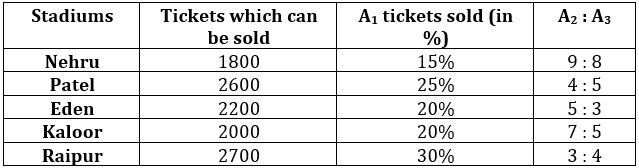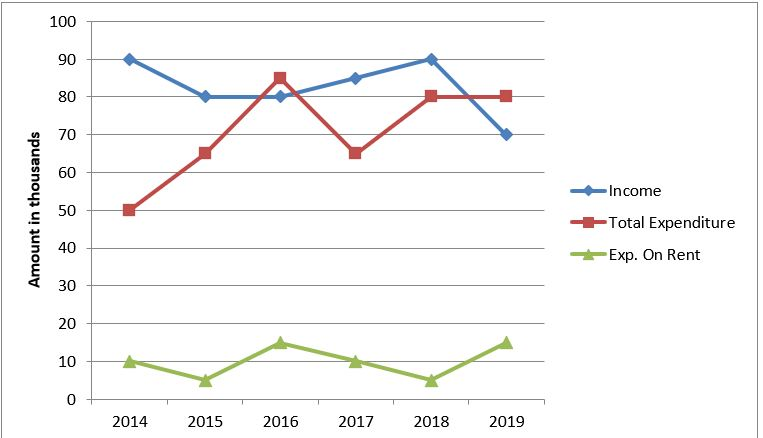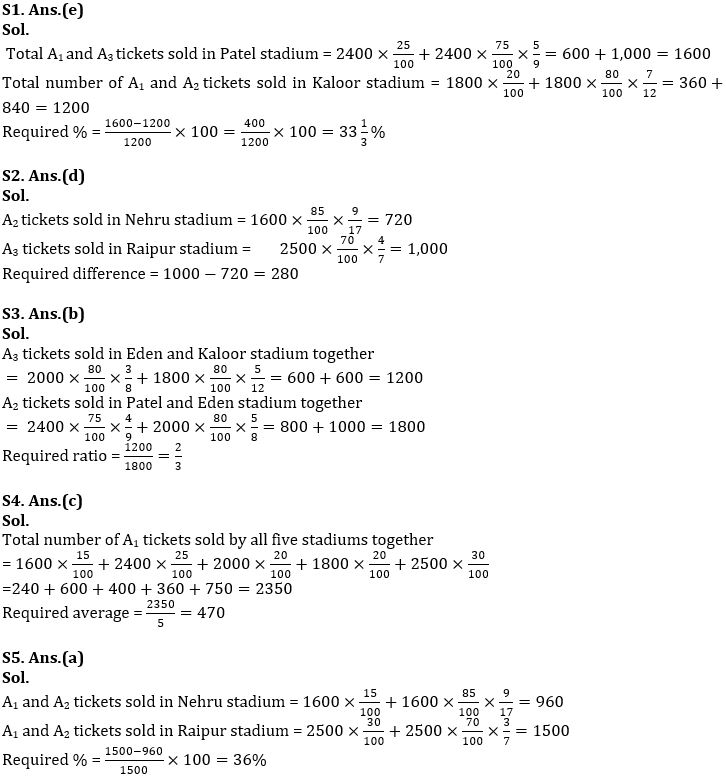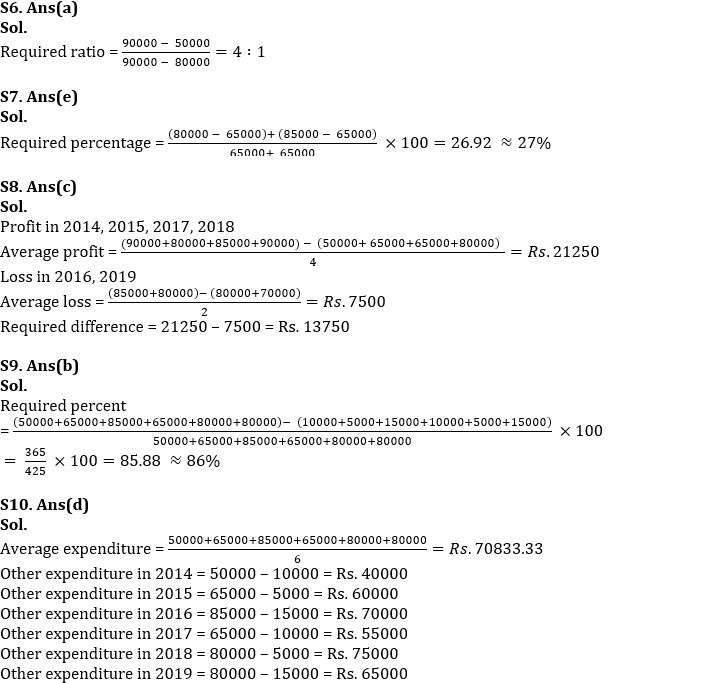Latest Banking jobs   »

# Quantitative Aptitude Quiz For IBPS RRB PO/Clerk Mains 2022- 22nd September

Directions (1- 5): Table given below shows total number of tickets which were estimated to be sold in five stadiums. Out of total tickets sold, percentage of A1 tickets sold, and ratio between A2 and A3 is given. Study the data carefully and answer the following questions.Note: – 200 tickets remain unsold in each stadium
Tickets which can be sold = A1 tickets + A2 tickets + A3 tickets + Unsold tickets

Q1. Total A1 and A3 tickets sold in Patel stadium is what percent more than total number of A1 and A2 tickets sold in Kaloor stadium?
(a) 16⅔%
(b) 50%
(c) 66⅔%
(d) 25%
(e) 33⅓%

Q2. Total A2 tickets sold in Nehru stadium is how much less than ¬A3 tickets sold in Raipur stadium?
(a) 220
(b) 240
(c) 260
(d) 280
(e) 300

Q3. Find the ratio between total A3 tickets sold in Eden and Kaloor stadium together to total A2 tickets sold in Patel and Eden stadium?
(a) 3 : 2
(b) 2 : 3
(c) 20 :23
(d) 23 : 20
(e) 8 : 9

Q4. Find the average number of total A1 tickets sold by all five stadiums together?
(a) 514
(b) 490
(c) 470
(d) 450
(e) 430

Q5. A1 and A2 tickets sold in Nehru stadium is what percent more/less than A1 and A2 tickets sold in Raipur stadium?
(a) 36%
(b) 64%
(c) 56.25%
(d) 43.75%
(e) 28%

Directions (6-10):- Given line graph shows the income & expenditure of a person over 6 years. Study the graph carefully and answer the following questions.NOTE:-
Profit = Income – Expenditure;
Loss = Expenditure – Income
Profit % = (profit/expenditure) * 100
Loss % = (loss/expenditure) * 100
Total Expenditure = Expenditure on Rent + Other Expenditure

Q6. What is the ratio of profit in 2014 to that of 2018?
(a) 4 : 1
(b) 5 : 8
(c) 16 : 17
(d) 6 : 1
(e) 12 : 17

Q7. What is the profit percentage in year 2015 and 2017 together? (in approx.)
(a) 22%
(b)24%
(c) 25%
(d) 29%
(e) 27%

Q8. What is the difference of average profit and average loss?
(a) Rs. 60000
(b) Rs. 27500
(c) Rs. 13750
(d) Rs. 11000
(e) Rs. 10625

Q9. Total other expenditure is what percent of total expenditure? (approx.)
(a) 84%
(b) 86%
(c) 88%
(d) 90%
(e) 92%

Q10. For how many years the other expenditure is more than the average expenditure?
(a) 3
(b) 5
(c) 4
(d) 1
(e) 2

Solutions#### Congratulations!Download Hindu Review of October 2021: Free PDF